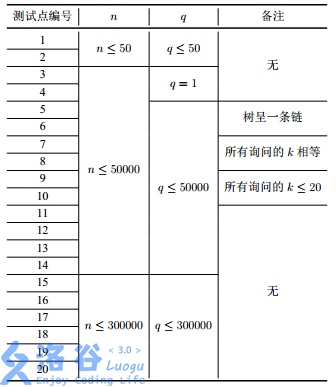# 图论 | 最小生成树 | 动态规划 | 线段树 | 主席树 | 20190710考试：解题报告

## Problem#1. 抓蛇 Snake

### Sample Input

6 2
7 9 8 2 3 2


### Sample Output

3


### 分析

$f[i][j]=min(f[i][j],\;f[k][j-1]+r[k+1][i])$

$r[k+1][j]=max[k+1][j]\times(j-(k+1)+1)-sum[k+1][j]$ ，其中 $sum[k+1][j]$ 为区间和

$f[i][j][k]=min(f[i][j][k]，f[i-1][j-1][k’])+a[k]-a[i]\quad(k’\in[1，n]，a[k]\geq a[i])$

### Codes

#include <cstdio>
#include <cstring>
#include <queue>
#include <iostream>
#include <vector>
#include <cmath>
#include <algorithm>
#define maxn 401
using namespace std;
template<typename t>inline void fcin(t &x){
int sign=1; x=0; char op=getchar();
while(op<'0'||op>'9'){if(op=='-') sign=-1;op=getchar();}
while(op>='0'&&op<='9'){x=x*10+(op-48);op=getchar();}
x*=sign;
}
int f[maxn][maxn];
int a[maxn],n,k;
int main(){
#ifndef ONLINE_JUDGE
freopen("snake.in","r",stdin);
freopen("snake.out","w",stdout);
#endif
fcin(n);fcin(k);
memset(f,127,sizeof(f));
for(int i=1;i<=n;i++)
fcin(a[i]);
for(int i=1;i<=n;i++){
if(a[i]>=a) f[i]=a[i]-a;
f=min(f,f[i]);
}
for(int i=2;i<=n;i++){
memset(f[i&1],127,sizeof(f[i&1]));
for(int j=0;j<=k;j++)
for(int p=1;p<=n;p++){
if(a[p]<a[i]) continue;
f[i&1][j][p]=min(f[i&1][j][p],f[(i-1)&1][j][p]+a[p]-a[i]);
if(j>=1) f[i&1][j][p]=min(f[i&1][j][p],f[(i-1)&1][j-1]+a[p]-a[i]);
f[i&1][j]=min(f[i&1][j],f[i&1][j][p]);
}
}
printf("%d",f[n&1][k]);
return 0;
}


## Problem#2. 分组行走 walk

### Sample Input

3 2


### Sample Output

2019201769


### Codes

#include <cstdio>
#include <cstring>
#include <queue>
#include <iostream>
#include <vector>
#include <cmath>
#include <algorithm>
#define maxn 7502
#define maxm maxn*(maxn-1)
using namespace std;
typedef long long ll;
template<typename t>inline void fcin(t &x){
int sign=1; x=0; char op=getchar();
while(op<'0'||op>'9'){if(op=='-') sign=-1;op=getchar();}
while(op>='0'&&op<='9'){x=x*10+(op-48);op=getchar();}
x*=sign;
}
int n,k;
inline ll pets(int x,int y){
return ((ll)2019201913*x+(ll)2019201949*y)%2019201997;
}
int main(){
#ifndef ONLINE_JUDGE
freopen("walk.in","r",stdin);
freopen("walk.out","w",stdout);
#endif
fcin(n);fcin(k);
printf("%lld",pets(k-1,n));
return 0;
}


## Problem#3. 谈笑风生 talk

### Description

• 设 a 和 b 为 T 中的两个不同节点。如果 a 是 b 的祖先，那么称“a 比 b 不知道高明到哪里去了”。

• 设 a 和 b 为 T 中的两个不同节点。如果 a 与 b 在树上的距离不超过某个给定常数 x，那么称“a 与 b 谈笑风生”。

1. a、 b 和 c 为 T 中三个不同的点，且 a 为 p 号节点；
2. a 和 b 都比 c 不知道高明到哪里去了；
3. a 和 b 谈笑风生。这里谈笑风生中的常数为给定的 k。

### Sample Input

5 3
1 2
1 3
2 4
4 5
2 2
4 1
2 3


### Sample Output

3
1
3


### Hint### Codes

#include <cstdio>
#include <cstring>
#include <queue>
#include <iostream>
#include <vector>
#include <cmath>
#include <algorithm>
#define maxn (300001*10)
#define maxm (maxn*2)
using namespace std;
typedef long long ll;
template<typename t>inline void fcin(t &x){
int sign=1; x=0; char op=getchar();
while(op<'0'||op>'9'){if(op=='-') sign=-1;op=getchar();}
while(op>='0'&&op<='9'){x=x*10+(op-48);op=getchar();}
x*=sign;
}
int newp,root[maxn];
struct TT{
struct TTNode{
int LC,RC; ll VAL;
#define lc(x) T[x].LC
#define rc(x) T[x].RC
#define val(x) T[x].VAL
#define mid ((L+R)>>1)
}T[maxn<<1];
inline void init(int &x,int y,int L,int R,int pos,int d){
x=++newp;val(x)=val(y)+d;
if(L==R) return;
lc(x)=lc(y);rc(x)=rc(y);
if(pos<=mid) init(lc(x),lc(y),L,mid,pos,d);
else init(rc(x),rc(y),mid+1,R,pos,d);
}
ll res(int k,int L,int R,int x,int y){
if(!k) return 0; y=(y>R)?R:y;
if(x==L && y==R) return val(k);
if(y<=mid) return res(lc(k),L,mid,x,y);
else if(x>mid) return res(rc(k),mid+1,R,x,y);
else return res(lc(k),L,mid,x,mid)+res(rc(k),mid+1,R,mid+1,y);
}
}st;int ref[maxn],dep[maxn],siz[maxn];
int tot,n,s,dfn,ld[maxn],rd[maxn];
}
void dfs(int u,int fu){
ld[u]=++dfn;ref[dfn]=u;
if(to[i]!=fu){
dep[to[i]]=dep[u]+1;
dfs(to[i],u);siz[u]+=siz[to[i]]+1;
}
rd[u]=dfn;
}
int main(){
#ifndef ONLINE_JUDGE
freopen("talk.in","r",stdin);
freopen("talk.out","w",stdout);
#endif
fcin(n);fcin(s);int x,y;
for(int i=1;i<n;i++){
fcin(x);fcin(y);
} dfs(1,0); ll ans=0;
for(int i=1;i<=n;i++) dep=max(dep,dep[i]);
for(int i=1;i<=n;i++)
st.init(root[i],root[i-1],0,dep,dep[ref[i]],siz[ref[i]]);
while(s--){
fcin(x);fcin(y);ans=0;
ans+=(ll)siz[x]*min(dep[x],y);
ans+=st.res(root[rd[x]],0,dep,dep[x]+1,dep[x]+y);
ans-=st.res(root[ld[x]-1],0,dep,dep[x]+1,dep[x]+y);
printf("%lld\n",ans);
}
return 0;
}